#### 1. In a 10-km race, Grace ran the first 2 km in     1 5 h, the next 6 km at a speed of 9 km /h and the last stretch at 12 km/h. What was her average speed for the run?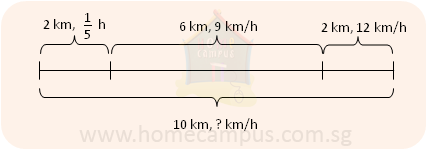Total distance  =  10 km

Let's calculate the total time taken:
Time taken for the first 2 km  -->
 1 5
h
Time taken for the next 6 km  -->
 6 9
h  =
 2 3
h
Time taken for the last 2 km  -->
 2 12
h  =
 1 6
h
Total time taken  =
 1 5
h  +
 2 3
h  +
 1 6
h  =
 31 30
h

Average speed  =  10 km  ÷
 31 30
h  =  10 km  ×
 30 31
h  =  9.67 km/h

Her average speed for the run was 9.67 km/h.

#### 2. Bella is taking an adventurous trip through a jungle. First, she trekked for 30 minutes into the thick jungle at an average speed of 6 km/h. Next, she took a boat to cross a lake which rode her at an average speed of 20 km/h for 12 minutes. For the last part of her trip, she hiked for 2 hours at an average speed of 4 km/h. Find the total distance she covered on her trip.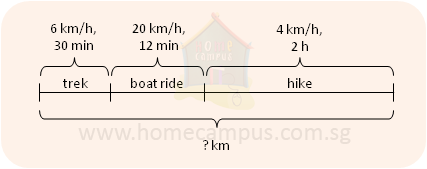Distance travelled during the first part
Average speed  =  6 km/h
Time taken  =  30 min  =
 1 2
h
Distance travelled  =  6 km/h  ×
 1 2
h  =  3 km
Distance travelled during the second part
Average speed  =  20 km/h
Time taken  =  12 min  =
 1 5
h
Distance travelled  =  20 km/h  ×
 1 5
h  =  4 km
Distance travelled during the last part
Average speed  =  4 km/h
Time taken  =  2 h  =
 1 5
h
Distance travelled  =  4 km/h  ×  2 h  =  8 km
Total distance travelled  =  3 km  +  4 km  +  8 km  =  15 km

She covered a total distance of 15 km on her trip.

#### 3. Eric walked at an average pace of 65 m/min for the first half of the distance to his friend's house. Calculate his average speed for the second half of the distance if he took a total of 18 minutes to reach his friend's house which was 1.3 km away.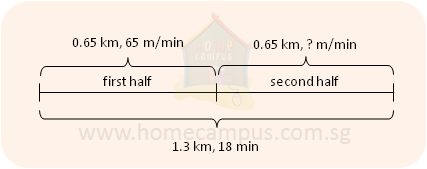For the first half
Distance covered  =  1.3 km  ÷  2  =  0.65 km  =  650 m
Average speed  =  65 m/min
Time taken  =  650 m  ÷  65 m/min  =  10 min
For the second half
Distance covered  =  650 m
Time taken  =  18 min  −  10 min  =  8 min
Average speed  =  650 m  ÷  8 min  =  81.25 m/min
His average speed for the second half of the distance was 81.25 m/min.

#### 4. Sally and Molly started from the same point at the same time in two different cars and drove on the same route to reach the same destination at average speeds of 40 km/h and 45 km/h respectively. If the route was 270 kilometres long and if Molly arrived at the destination at 5:30 pm, when did Sally arrive there?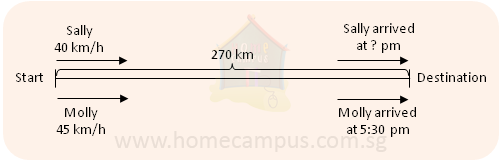Time taken by Sally
=  270 km  ÷  40 km/h
=  6.75 h
=  6 h  +  0.75 h
=  6 h  +  (0.75 × 60) min
=  6 h  +  45 min
=  6 h 45 min

Time taken by Molly
=  270 km  ÷  45 km/h
=  6 h

Sally took 45 min more than Molly.
45 min after 5:30 pm is 6:15 pm.
Sally arrived at the destination at 6:15 pm.

#### 5. Carol and Maya took part in a 20-km cycle race. Carol rode at an average speed of 22 km/h while Maya rode at an average speed of 25 km/h. How far apart will the two girls be when Maya has covered half the distance?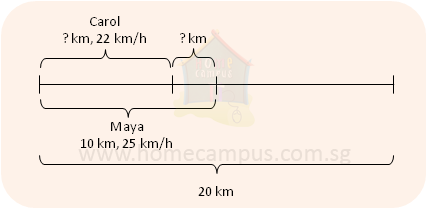Time taken by Maya to cover half the distance  =  10 km  ÷  25 km/h  =
 2 5
h

Distance covered by Carol in
 2 5
h  =  22 km/h  ×
 2 5
h  =  8.8 km

Distance between Maya and Carol after
 2 5
h  =  10 km  −  8.8 km  =  1.2 km

The girls will be 1.2 km apart when Maya has covered half the distance.

#### 6. James and Lily, who were standing 29.25 km apart, started running towards each other at 0915. James ran at an average speed of 5.5 km/h while Lily ran at an average speed of 7.5 km/h. a)   At what time did they meet? b)   How much distance had Lily run when they both met?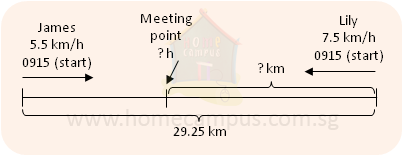a)   Distance covered by James in 1 h  =  5.5 km
Distance covered by Lily in 1 h  =  7.5 km
Total distance covered by James and Lily in 1 h  =  5.5 km  +  7.5 km  =  13 km

Time taken to cover 13 km  =  1 h

Time taken to cover 29.25 km
=  2.25 h
=  2 h  +  0.25 h
=  2 h  +  (0.25 × 60) min
=  2 h + 15 min
=  2 h 15 min

2 h 15 min after 0915 is 1130.

James and Lily met at 1130.

b)   Distance run by Lily in 2 h 15 min
=  7.5 km/h  ×  (2 h 15 min)
=  7.5 km/h  ×  2.25 h
=  16.875 km

Lily had run 16.875 km when they both met.

#### 7. What is the speed at which the Earth revolves around the Sun? Note: Assume the Earth's orbit around the Sun to be circular instead of elliptical.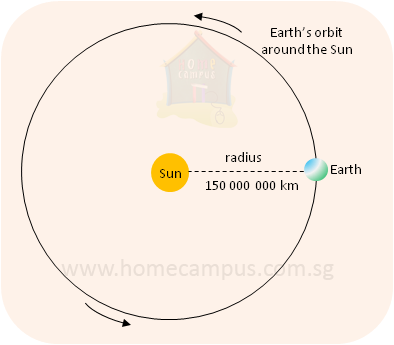The radius is the average distance of the Earth from the Sun
≈  150 000 000 km
=  150 million kilometres
Time taken by the Earth to make one revolution around the Sun  =  1 year

Distance travelled by the Earth around the Sun in 1 year
=  the orbit of the Earth
=  circumference of the circle or the orbit
=  2  ×  π  ×  radius
=  2  ×  3.14  ×  150 000 000 km
=  942 000 000 km

Speed  =
 Distance travelled Time taken

 942 000 000 km 1 year

 942 000 000 km 365 days

 2 580 822 km 1 day

 2 580 822 km 24 hours

=  107 534 km/h
≈  100 000 km/h

So, the Earth revolves around the sun at a speed of about 100 000 km/h.
At this speed, it will take only 9 minutes to travel from Singapore to New York!